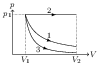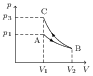# Isothermal and Adiabatic Processes

## Problems from IIT JEE

Problem (IIT JEE 2011): 5.6 litre of helium gas at STP is adiabatically compressed to 0.7 litre. Taking the initial temperature to be $T_1$, the work done in the process is,

1. $\frac{9}{8}RT_{1}$
2. $\frac{3}{2}RT_{1}$
3. $\frac{15}{8}RT_{1}$
4. $\frac{9}{2}RT_{1}$

Solution: For an ideal gas, $pV=nRT$. In an adiabatic process, $TV^{\gamma-1}=\text{constant}$, and the work done by the gas is given by, \begin{alignat}{2} W=\frac{p_1 V_1 -p_2 V_2}{\gamma -1}=\frac{nRT_1 -nRT_2}{\gamma -1}=\frac{nRT_1}{\gamma -1}\left[1-\left(\frac{V_1}{V_2}\right)^{\gamma-1}\right], \nonumber \end{alignat} where $V_1=5.6\;\mathrm{litre}$ and $V_2=0.7\;\mathrm{litre}$ are initial and final volume of the gas. Now, 5.6 litre at STP gives $n=5.6/22.4=1/4\;\mathrm{mol}$. Helium, being a monoatomic gas, has $\gamma=5/3$. Substitute these values to get $W=-\frac{9}{8}RT_1$. Negative sign indicates that work is done on the gas.

Problem (IIT JEE 2010): Starting with the same initial conditions, an ideal gas expands from volume $V_1$ to $V_2$ in three different ways. The work done by the gas is $W_1$ if the process is purely isothermal, $W_2$ if purely isobaric and $W_3$ if purely adiabatic, then,

1. $W_2 > W_1 > W_3$
2. $W_2 > W_3 > W_1$
3. $W_1 > W_2 > W_3$
4. $W_1 > W_3 > W_2$

Solution: Let $p_1$, $V_1$, and $T_1$ be initial pressure, volume, and temperature of the gas. For an ideal gas, $p_1V_1=nRT_1$. The work done by the gas in isothermal, isobaric, and adiabatic processes are given by, \begin{align} W_1&=p_1V_1\ln \left(\frac{V_2}{V_1}\right), \nonumber\\ W_2&=p_1(V_2-V_1), \nonumber\\ W_3&=\frac{p_1V_1-p_2V_2}{\gamma-1}=\frac{p_1V_1}{\gamma-1}\left[1-\left(\frac{V_1}{V_2}\right)^{\gamma-1}\right], \nonumber \end{align} where $p_2$ and $V_2$ are final pressure and volume. If relationship among $W_1$, $W_2$, and $W_3$ holds in general then it should hold good for any special case, say $V_2=2V_1$ and $\gamma=2$. In this case, $W_1=0.693 p_1 V_1$, $W_2=p_1 V_1$, and $W_3=0.5 p_1 V_1$, giving $W_2 > W_1 > W_3$.Aliter: The isothermal, isobaric, and adiabatic processes starting at the same initial point ($p_1,V_1$) and having same final volume $V_2$ are drawn in the figure. The work done in a process is equal to the area under the curve on $p\text{-}V$ diagram. It is clear from the diagram that $W_2 > W_1 > W_3$.

Problem (IIT JEE 2004): An ideal gas expands isothermally from a volume $V_1$ to $V_2$ and then compressed to original volume $V_1$ adiabatically. Initial pressure is $p_1$ and final pressure is $p_3$. The total work done is $W$. Then,

1. $p_3 > p_1$, $W > 0$
2. $p_3 < p_1$, $W < 0$
3. $p_3 > p_1$, $W < 0$
4. $p_3 = p_1$, $W = 0$

Solution:On a $p\text{-}V$ diagram, magnitude of slope of an adiabatic process is greater than that of an isothermal process (adiabatic process is more steeper). This fact is applied at the point B to draw the isothermal process AB and the adiabatic process BC (see figure). Note that slope is negative in both cases because it is defined as tangent ($\tan$) of an angle from $x$-axis measured in anti-clockwise direction. From the graph $p_3>p_1$. The work done by the gas is area under the $p\text{-}V$ curve taken positive for expansion and negative for contraction. Thus, $W_\text{AB}$ is positive and $W_\text{BC}$ is negative and $|W_\text{BC}|>|W_\text{AB}|$. Thus, total work done $W=W_\text{AB}+W_\text{BC}<0$. The readers are encouraged to find expressions for $p_3$ and $W$ in terms of given parameters. Hint: $p_3=p_1\left(V_2/V_1\right)^{\gamma-1}$, $W_\text{AB}=p_1V_1\ln\frac{V_2}{V_1}$, and $W_\text{BC}=\frac{p_1V_1}{\gamma-1}\left(1-(V_2/V_1)^{\gamma-1}\right)$.